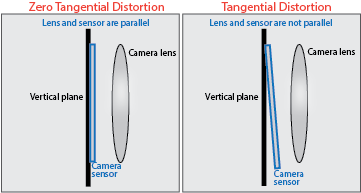# cameraIntrinsics

Object for storing intrinsic camera parameters

## Description

Store information about a camera’s intrinsic calibration parameters, including the lens distortion parameters.

## Creation

### Syntax

``intrinsics = cameraIntrinsics(focalLength,principalPoint,imageSize)``
``intrinsics = cameraIntrinsics(focalLength,principalPoint,imageSize,Name,Value)``

### Description

example

````intrinsics = cameraIntrinsics(focalLength,principalPoint,imageSize)` returns a camera intrinsics object that contains the focal length and the camera's principal point. The three input arguments set the FocalLength, PrincipalPoint, and ImageSize properties, respectively.```
````intrinsics = cameraIntrinsics(focalLength,principalPoint,imageSize,Name,Value)` sets additional properties using one or name-value pairs. Enclose each property name in quotes.For example, ```intrinsics = cameraIntrinsics(focalLength,principalPoint,imageSize,"RadialDistortion",[0 10])``` sets the radial lens distortion property, `RadialDistortion`, as the vector `[0 10]`.```

## Properties

expand all

Focal length in x and y, stored as a 2-element vector [fx fy] in pixels.

fx = F * sx

fy = F * sy

F is the focal length in world units, typically in millimeters. sx and sy are the number of pixels per world unit in the x and y direction respectively. Thus, fx and fy are in pixels.

The focal length F influences the angle of view and thus affects the area of the scene that appears focused in an image. For a fixed subject distance:

• A short focal length offers a wide angle of view allowing to capture large area of the scene under focus. It emphasizes both the subject and the scene background.

• A long focal length offers a narrow angle of view, thus reducing the area of the scene under focus. It emphasizes more on the subject and restricts the amount of background from being captured.

You cannot set the value of `FocalLength` after you create the `cameraIntrinsics` object.

Optical center of camera, stored as a two-element vector [cx cy] in pixels. The vector contains the coordinates of the optical center of the camera.

You cannot set the value of `PrincipalPoint` after you create the `cameraIntrinsics` object.

Image size produced by the camera, stored as a two-element vector, [mrows ncols].

You cannot set the value of `ImageSize` after you create the `cameraIntrinsics` object.

Radial lens distortion, specified as a two-element vector, [k1 k2], or a three-element vector, [k1 k2 k3]. The elements k1, k2, and k3 are radial distortion coefficients.

Radial distortion is the displacement of image points along radial lines extending from the principal point.

• As image points move away from the principal point (positive radial displacement), image magnification decreases and a pincushion-shaped distortion occurs on the image.

• As image points move toward the principal point (negative radial displacement), image magnification increases and a barrel-shaped distortion occurs on the image.The camera parameters object calculates the radial distorted location of a point. You can denote the distorted points as (xdistorted, ydistorted), as follows:

xdistorted = x(1 + k1*r2 + k2*r4 + k3*r6)

ydistorted = y(1 + k1*r2 + k2*r4 + k3*r6)

 x, y are undistorted pixel locations k1, k2, and k3 are radial distortion coefficients of the lens r2 = x2 + y2
Typically, two coefficients are sufficient. For severe distortion, you can include k3. The undistorted pixel locations appear in normalized image coordinates, with the origin at the optical center. The coordinates are expressed in world units.

Tangential distortion coefficients, specified as a 2-element vector, [p1 p2]. Tangential distortion occurs when the lens and the image plane are not parallel.The camera parameters object calculates the tangential distorted location of a point. You can denote the distorted points as (xdistorted, ydistorted), as follows:

xdistorted = x + [2 * p1 * x * y + p2 * (r2 + 2 * x2)]

ydistorted = y + [p1 * (r2 + 2*y2) + 2 * p2 * x * y]

 x, y are undistorted pixel locations p1 and p2 are tangential distortion coefficients of the lens r2 = x2 + y2
The undistorted pixel locations appear in normalized image coordinates, with the origin at the optical center. The coordinates are expressed in world units.

Camera axes skew, specified as a numeric scalar. If the x and the y axes are exactly perpendicular, then the skew must be `0`.

Camera intrinsic matrix, specified as a 3-by-3 matrix. The matrix has the format

`$\left[\begin{array}{ccc}{f}_{x}& s& {c}_{x}\\ 0& {f}_{y}& {c}_{y}\\ 0& 0& 1\end{array}\right]$`

The coordinates [cx cy] represent the optical center (the principal point), in pixels. When the x and y axis are exactly perpendicular, the skew parameter, s, equals `0`.

fx = F*sx

fy = F*sy

 F is the focal length in world units, typically expressed in millimeters. sx and sy are the number of pixels per world unit in the x and y direction respectively. fx and fy are expressed in pixels.

## Examples

collapse all

Define camera parameters without lens distortion or skew.

Specify the focal length and principal point in pixels.

```focalLength = [800 800]; principalPoint = [320 240]; imageSize = [480 640];```

Create a camera intrinsics object.

`intrinsics = cameraIntrinsics(focalLength,principalPoint,imageSize)`
```intrinsics = cameraIntrinsics with properties: FocalLength: [800 800] PrincipalPoint: [320 240] ImageSize: [480 640] RadialDistortion: [0 0] TangentialDistortion: [0 0] Skew: 0 K: [3×3 double] ```

## Version History

Introduced in R2017a

expand all